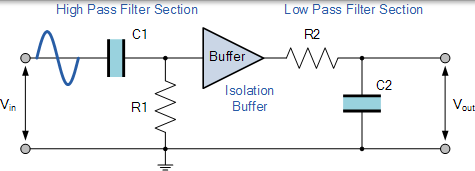# 无源带通滤波器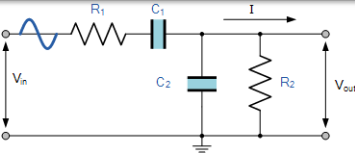### 带通滤波器电路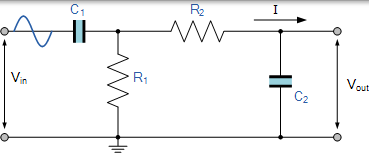### 二阶带通滤波器的频率响应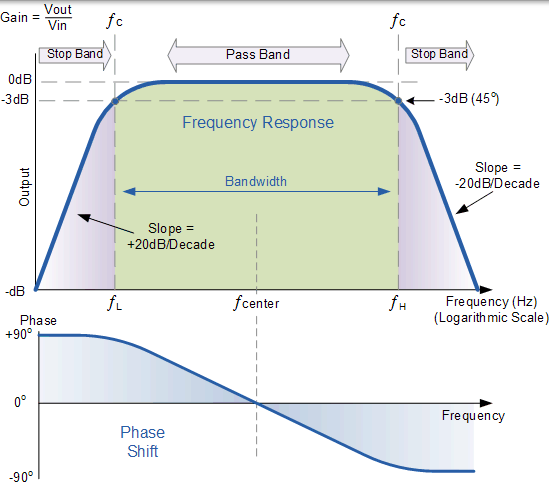$$f _ { C } = \frac { 1 } { 2 \pi R C } H z$$

## 带通滤波器示例 No1

### 高通滤波器阶段

$$C _ { 1 } = \frac { 1 } { 2 \pi f _ { L } R } = \frac { 1 } { 2 \pi \times 1,000 \times 10,000 } = 15.9 n F$$

### 低通滤波器阶段

$$C _ { 2 } = \frac { 1 } { 2 \pi f _ { H } R } = \frac { 1 } { 2 \pi \times 30,000 \times 10,000 } = 530 p F$$

### 完成带通滤波器电路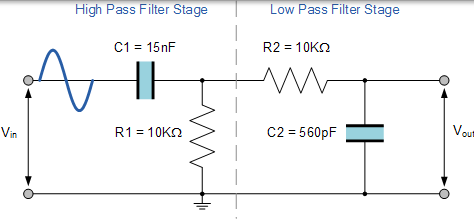## 带通滤波器谐振频率

### 中心频率方程

$$f r = \sqrt { f _ { L } x f _ { H } }$$

• ƒr 是谐振或中心频率
• ƒL 是下 -3dB 截止频率点
• ƒH 是上 -3db 截止频率点

$$f r = \sqrt { f _ { L } \times f _ { H } } = \sqrt { 1,060 \times 28,420 } = 5,48 k H z$$

## 带通滤波器摘要

### 缓冲单个滤波阶段